Diagnostics Based on Weighted Residuals

The vector of weighted Schoenfeld residuals,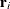, is computed as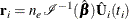where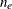is the total number of events and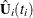is the vector of Schoenfeld residuals at the event time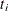. The components ofare output to the WTRESSCH= variables.

The weighted Schoenfeld residuals are useful in assessing the proportional hazards assumption. The idea is that most of the common alternatives to the proportional hazards can be cast in terms of a time-varying coefficient model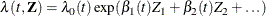where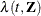and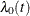are hazard rates. Let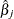and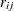be the jth component of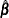and, respectively. Grambsch and Therneau (1994) suggest using a smoothed plot of (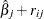) versusto discover the functional form of the time-varying coefficient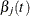. A zero slope indicates that the coefficient is not varying with time.

The weighted score residuals are used more often than their unscaled counterparts in assessing local influence. Let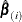be the estimate ofwhen the ith subject is left out, and let. The jth component of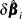can be used to assess any untoward effect of the ith subject on. The exact computation ofinvolves refitting the model each time a subject is omitted. Cain and Lange (1984) derived the following approximation of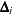as weighted score residuals: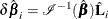Here,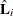is the vector of the score residuals for the ith subject. Values ofare output to the DFBETA= variables. Again, when the counting process MODEL specification is used, the DFBETA= variables contain the component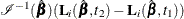, where the score process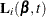is defined in the section Residuals. The vectorfor the ith subject can be obtained by summing these components within the subject.

Note that these DFBETA statistics are a transform of the score residuals. In computing the robust sandwich variance estimators of Lin and Wei (1989) and Wei, Lin, and Weissfeld (1989), it is more convenient to use the DFBETA statistics than the score residuals (see Example 67.10).